# Vector equation

Let’s v = (1, 2, 1), u = (0, -1, 3) and w = (1, 0, 7) . Solve the vector equation c1 v + c2 u + c3 w = 0 for variables c1 c2, c3 and decide weather v, u and w are linear dependent or independent

Result

c1 = (Correct answer is: )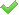c2 = (Correct answer is: 2 c1)c3 = (Correct answer is: -c1)#### Solution:

$c_{1} \cdot \ 1+c_{2} \cdot \ 0+c_{3} \cdot \ 1=0 \ \\ c_{1} \cdot \ 2+c_{2} \cdot \ (-1)+c_{3} \cdot \ 0=0 \ \\ c_{1} \cdot \ 1+c_{2} \cdot \ 3+c_{3} \cdot \ 7=0 \ \\ \ \\ c_{1} + c_{3}=0 \ \\ 2 \ c_{1} - c_{2}=0 \ \\ c_{1} + 3 \ c_{2} + 7 \ c_{3}=0 \ \\ \ \\ c_{1}=any \ \\ c_{2}=2 \ c_{1} \ \\ c_{3}=-c_{1}$
$c_{2}=2 \ c_{1}$
$c_{3}=-c_{1}$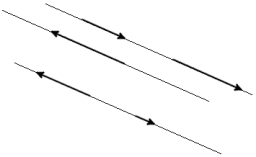Our examples were largely sent or created by pupils and students themselves. Therefore, we would be pleased if you could send us any errors you found, spelling mistakes, or rephasing the example. Thank you!

Please write to us with your comment on the math problem or ask something. Thank you for helping each other - students, teachers, parents, and problem authors.Matematik
linearly independentMath student
c1 = (Correct answer is: ) OKTips to related online calculators
For Basic calculations in analytic geometry is helpful line slope calculator. From coordinates of two points in the plane it calculate slope, normal and parametric line equation(s), slope, directional angle, direction vector, the length of segment, intersections the coordinate axes etc.
Two vectors given by its magnitudes and by included angle can be added by our vector sum calculator.
Do you have a linear equation or system of equations and looking for its solution? Or do you have quadratic equation?

## Next similar math problems:

• Vector perpendicularFind the vector a = (2, y, z) so that a⊥ b and a ⊥ c where b = (-1, 4, 2) and c = (3, -3, -1)
• Parallel and orthogonal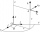I need math help in this problem: a=(-5, 5 3) b=(-2,-4,-5) (they are vectors) Decompose the vector b into b=v+w where v is parallel to a and w is orthogonal to a, find v and w
• Vector v4Find the vector v4 perpendicular to vectors v1 = (1, 1, 1, -1), v2 = (1, 1, -1, 1) and v3 = (0, 0, 1, 1)
• Scalar product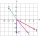Calculate the scalar product of two vectors: (2.5) (-1, -4)
• Coordinates of vector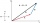Determine the coordinate of a vector u=CD if C(19;-7) and D(-16;-5)
• Vector - basic operationsThere are given points A [-9; -2] B [2; 16] C [16; -2] and D [12; 18] a. Determine the coordinates of the vectors u=AB v=CD s=DB b. Calculate the sum of the vectors u + v c. Calculate difference of vectors u-v d. Determine the coordinates of the vector w
• VectorDetermine coordinates of the vector u=CD if C[19;-7], D[-16,-5].
• Men, women and childrenOn the trip went men, women and children in the ratio 2:3:5 by bus. Children pay 60 crowns and adults 150. How many women were on the bus when a bus was paid 4,200 crowns?
• Ball gameRichard, Denis and Denise together scored 932 goals. Denis scored 4 goals over Denise but Denis scored 24 goals less than Richard. Determine the number of goals for each player.
• ParabolaFind the equation of a parabola that contains the points at A[6; -5], B[14; 9], C[23; 6]. (use y = ax2+bx+c)
• Stones 3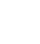Simiyu and Nasike each collected a number of stones in an arithmetic lesson. If Simiyu gave Nasike 5 stones, Nasike would have twice as many stones as Simiyu. If initially, Simiyu had five stones less than Nasike how many stones did each have?
• Null points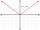Calculate the roots of the equation: ?
• Geometric sequence 5About members of geometric sequence we know: ? ? Calculate a1 (first member) and q (common ratio or q-coefficient)
• ChildrenThe group has 42 children. There are 4 more boys than girls. How many boys and girls are in the group?
• Warehouses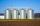In the three warehouses, a total of 70 tons of grain was stored. In the second warehouse was stored 8.5t less and in the third 3.5t more than in the first. How many tons of grain was stored in each warehouse?
• Linsys2Solve two equations with two unknowns: 400x+120y=147.2 350x+200y=144
• AP - basicsDetermine first member and differentiate of the the following sequence: a3-a5=24 a4-2a5=61